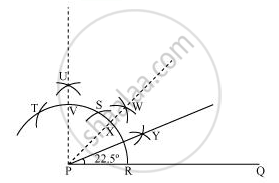# Construct the angles of the following measurement 22 1/2° - Mathematics

Construct the angles of the following measurement:- 22 1/2^@

#### Solution

The below given steps will be followed to construct an angle of 22 1/2@

(1) Take the given ray PQ. Draw an arc of some radius, taking point P as its centre, which intersects PQ at R.

(2) Taking R as centre and with the same radius as before, draw an arc intersecting the previously drawn arc at S.

(3) Taking S as centre and with the same radius as before, draw an arc intersecting the arc at T (see figure).

(4) Taking S and T as centre, draw an arc of same radius to intersect each other at U.

(5) Join PU. Let it intersect the arc at point V.

(6) From R and V, draw arcs with radius more than 1/2RV to intersect each other at W. Join PW.

(7) Let it intersect the arc at X. Taking X and R as centre and radius more than 1/2RX, draw arcs to intersect each other at Y.

Joint PY which is the required ray making 22 1/2@ with the given ray PQ.Concept: Basic Constructions
Is there an error in this question or solution?

#### APPEARS IN

NCERT Class 9 Maths
Chapter 11 Constructions
Exercise 11.1 | Q 3.2 | Page 191